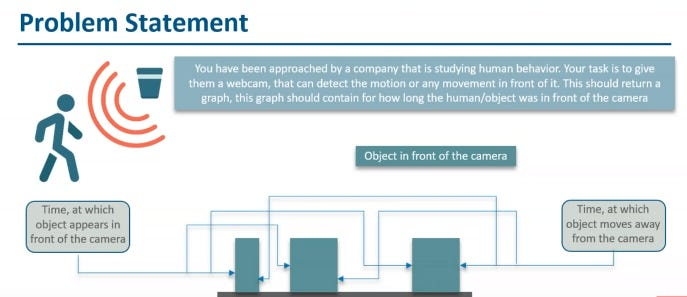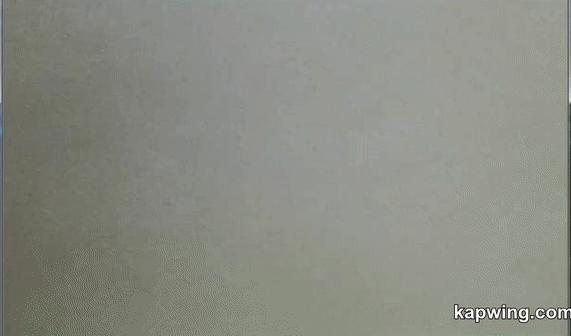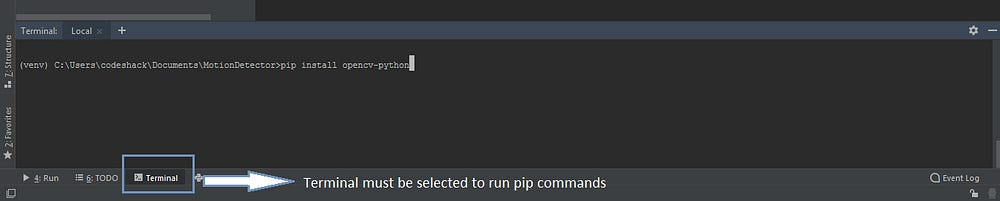# Creating Motion Object Detector Using OpenCV and Python on Windows

Source: Deep Learning on Medium

We often come to a problem where we need to detect moving objects from a camera. Ever thought how can we do it ? And how complex it is?

Well if you ask me, when I started, terms like using a camera (Computer Vision), coding in python, Deep learning, Algorithms, Data models… everything sounded complex. But when I actually started with it by reading various documentations of available libraries and tutorials on Youtube, it seemed very easy. Almost everything is readily available, all you need is to put different components/libraries and wireframe into one single application to achieve your goal.Camera detecting moving persion/object and recording its in and out time and then plotting a graph.

### How it will look like ?Forgive me for testing it with my hand. It could be any object that’s moving in front camera.

What all you need ?
Python — Installed in Windows
PIP Installer (Mostly come along with Python installation package)
OpenCv — Python : For reading video/frames from camera
NumPy : For converting pixels captured from OpenCV into Numpy Array
Pandas : For creating data frames and Loggin the timing into a .csv file
Bokeh : For plotting the graphing using data frames created by Pandas
And it the end of course we need a Python IDE, well I am using PyCharm for this.

***Ensure you select “Add Python to PATH” checkbox
***Ensure you chose “Install Now” option to install all supported libraries by default.

2. After Installation ensure Python is working as expected in your system. Open command prompt and run this command: “Python — — version”, it must display the version of Python.

4. Post installation open PyCharm and Create a new Project.
5.Then Install the required packages from Terminal using PIP Commands:
OpenCV-Python : “pip install opencv-python
NumPy : “pip install numpy
Pandas: “pip install pandas
Bokeh: “pip install bokehIn PyCharm at bottom you will find Terminal, where you can run all python or PIP commands.

So how we are going to achieve it ?
All we need is to detect moving objects in a video stream(source being camera here).
What is a video ? It’s nothing but multiple images or frames which are displayed very quickly.
So using OpenCV, we will capture the frames and will loop through each frame so that it appears like a video.
To detect the moving objects, we will store the first image as the base frame and then with each further frames we will keep on substracting the frames, so that if at any point of time there is a difference b/w the two frames it means there is a new object in our frame.

Then we will detect that new object in our frame and will highlight in into the windows by drawing a rectange around it.
To increase the efficiency, we would convert each frame to gray scale and then Gaussian blur image.
Also a threshhold is defined to remove minor deviations in the frame, e.g., shadows, flies, etc as we are really not interested in detectiting those as our desired Objects.

Also we will log the time the object enters our frame and leaves our frame as Start and end timings in our dataframe using Pandas library.
Based on the dataframe created by Pandas, we will further plot a graph using Bokeh library.

The working code you can get from this Github repository : https://github.com/anshurajlive/motiondetector_opencv

Complex Code Explanations:

video = cv2.VideoCapture(0)
#creating a video object using OpenCv , 0 means the source of video would be the inbuilt camera of the device. (webcam in my case)

gray = cv2.cvtColor(frame, cv2.COLOR_BGR2GRAY)
gray = cv2.GaussianBlur(gray, (21, 21), 0)

#reading the video object and storing the individual frames inside the loop
#coverting the frame to gray scale
#converting the gray scale frame to Gaussian Blur image

if first_frame is None:
first_frame = gray
continue
#Storing the first frame in first_frame variable that will be used substract from further frames to get the objects.

delta_frame = cv2.absdiff(first_frame, gray)
th_delta = cv2.threshold(delta_frame, 60, 255, cv2.THRESH_BINARY)
th_delta = cv2.dilate(th_delta, None, iterations=0)
(cnts, _) = cv2.findContours(th_delta.copy(), cv2.RETR_EXTERNAL, cv2.CHAIN_APPROX_SIMPLE)
#substracting the subsequent gray scale frames in while loop with first_frame
#thresholding the delta_frame (difference in first and current frame) ~ If pixel value is greater than a threshold value, it is assigned one value (may be white), else it is assigned another value (may be black).
#dilating the thresh delta further to increse efficiency.
#finding the contours of the detected object in frame and storing in the variable cnts

for contour in cnts:
if cv2.contourArea(contour) < 10000:
continue
status = 1
(x, y, w, h) = cv2.boundingRect(contour)
cv2.rectangle(frame, (x, y), (x+w, y+h), (0, 255, 0), 3)
#looping through the contours and drawing a rectangle around the object

status_list.append(status)
status_list = status_list[-2:]
if status_list[-1] == 1 and status_list[-2] == 0:
times.append(datetime.datetime.now())
if status_list[-1] == 0 and status_list[-2] == 1:
times.append(datetime.datetime.now())
#changing the status value between 1 and 0 based on the object first enters and then leaves the frame
#then storing the current datetime first when the object enters the frame and then when it leaves the frame

cv2.imshow(‘Capturing’, frame)
key = cv2.waitKey(1)
if key == ord(‘q’):
break
#displaying the frame in window where we will detect the objects as well
#continue the while loop until user presses ‘q’ button on keyboard.

for i in range(0, len(times), 2):
df = df.append({“Start”: times[i], “End”: times[i+1]}, ignore_index=True)

df.to_csv(“Times_”+datetime.datetime.now().strftime(“%Y-%m-%d_%H%M%S”)+”.csv”)
video.release()
cv2.destroyAllWindows()
# storing te Start and End timing into the Pandas dataframe object “df”
# saving the the dataframe data into the .csv file in application rool folder
#releasing and destorying the objects at the end

df["Start_string”] = df[�["Start”]t.strftime(“%Y-%m-%d %H:%M:%S”)
df[“["End_string”]f[“En["End”]rftime(“%Y-%m-%d %H:%M:%S”)

cds=ColumnDataSource(df)

p=figure(x_axis_type=’datetime’, height=100, width=500, title=”Motion Graph”)
p.yaxis.minor_tick_line_color=None
p.ygrid.tickdesired_num_ticks=1

hover=HoverTool(tooltips=[(“Sta[("Start”, "@Start_string”), ("End”, "@End_string”)]dd_tools(hover)

q=p.quad(left=”Start”, right=”End”, bottom=0, top=1, color=”red”, source=cds)
output_file(“Graph”+datetime.datetime.now().strftime(“%Y-%m-%d_%H%M%S”)+”.html”)
show(p)
#Getting the data from Pandas dataframe object “df”
# plotting the graph on the axis after defining them